Science, Maths & Technology

### Become an OU studentIT: Information

Start this free course now. Just create an account and sign in. Enrol and complete the course for a free statement of participation or digital badge if available.

# 4.4.1 Voltage, current and resistance

Voltage (or, more correctly, electromotive force, emf – but I shall follow common practice and just say voltage) is a measure of the force with which electricity is 'pushed'. Nothing happens, however, unless there is an electric circuit, which is a path from one terminal of a voltage source (the battery, in this case) to the other, along which the electricity can flow (Figure 12).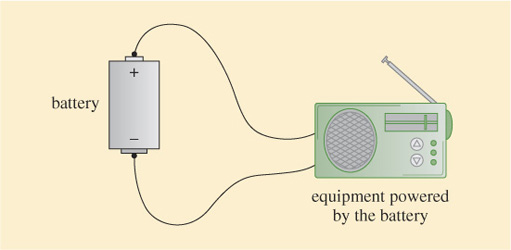Figure 12 An electric circuit

If there is an electric circuit, the rate at which electricity flows is determined by the nature of the circuit and the value of the battery's voltage. To quantify the rate at which electricity flows we need to know how much the circuit allows or resists the flow of electricity, and this is determined by a measure known as resistance.

If a circuit has a high resistance, little electricity flows for a given voltage. If it has a low resistance, a lot of electricity flows. Resistance is measured in units called ohms, and the rate of flow of electricity is measured in units called amps. The rate of electricity flow, which we call the electric current, in amps is calculated by dividing the battery voltage in volts by the circuit resistance in ohms.

That is: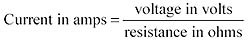Qualitatively, you might be able to see that this is plausible. The bigger the voltage (the stronger the push), the more current will flow. The bigger the resistance, on the other hand, the smaller the current.

The convention is to use symbols, i for current, v for voltage and r for resistance, so we write: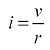For example, if a battery voltage is 3 volts and the circuit resistance is 10 ohms the current flowing is: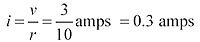The symbol for amps is a capital A, so this is written as 0.3 A. The symbol for volts is a capital V and for ohms is the capital Greek letter omega, Ω.

One amp is a fairly large current flow for electronic equipment, and quite often it is easier to work in units of 1/1000 of an amp which is 1 milliamp, written as 1 mA. Similarly, a resistance of 1 ohm is very small for electronic equipment and often resistance will be expressed in units of kilohms, kΩ, which are thousands of ohms.

## Activity 18

If the battery voltage is 1 V and the circuit resistance 1 kΩ, what is the current flow in mA?

### Discussion

1 kΩ=1000 Ω, so the current is given by: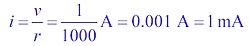The formula triangle that I introduced earlier can be used with the equation for v, i, and r This time because v is on the top of the fraction, v goes at the top of the triangle, with i and r in the bottom corners (again it does not matter which way around), resulting in a formula triangle as shown in Figure 13. The relationship between voltage, current and resistance represented by this triangle has a special name: it is known as Ohm's law. (Georg Simon Ohm, 1789–1854, was the German physicist who first described this relationship.)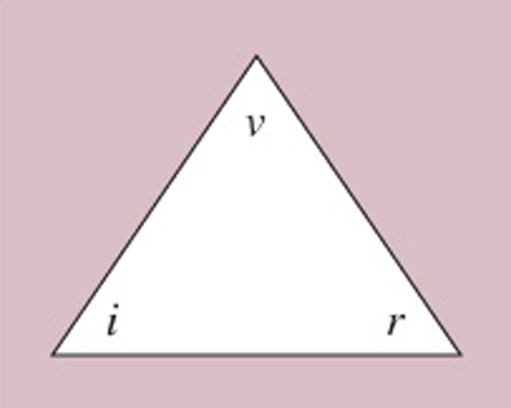Figure 13 The formula triangle for Ohm's law